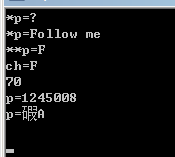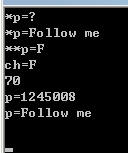•闪存
•博客
• 发言小组
• 投递新闻
• 提问博问
• 添加收藏
• 发布招聘
•文库

# 指向指针数据的指针。。。

0悬赏园豆：5 [已关闭问题] 关闭于 2013-05-13 00:37#include <stdio.h>

int main()
{
char **p;
int i;
char ch;
for (i=0;i<5;i++)
{
p=name+i;
printf("*p=%c\n",*p);//此处*p=name[i]字符串第一个字符的地址，输出最后两位数字的ascii码值
printf("*p=%s\n",*p);//此处*p=name[i]字符串第一个字符的地址，输出第i个字符串
printf("**p=%c\n",**p);//此处*p=name[i]字符串第一个字符，输出字符(**p)
printf("ch=%c\n",ch=**p);//此行是为了证明上面的结论的,输出同上
printf("%d\n",**p);//此处*p=name[i]字符串第一个字符的整形数字(ascii)，输出F=70；B=66；G=71；F=70；C=67
printf("p=%d\n",p);//name[i]字符串第一个字符的地址 (不同计器输出不同，但相邻值差4)
printf("p=%s\n",p);//这个直到现在我都还没揣摩出来(。。。。望知道者补充之。。)
printf("\n");
}
return 0;
}

1

printf("p=%s\n",p);//这个直到现在我都还没揣摩出来(。。。。望知道者补充之。。)0

56180825 | 园豆：1543 (小虾三级) | 2013-05-11 11:05

您需要登录以后才能回答，未注册用户请先注册